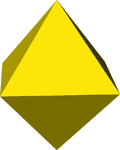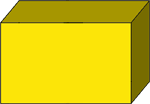# Intersecting planes

Intersecting planes are planes that intersect along a line.

## Polyhedra and intersecting planes

A polyhedron is a closed solid figure formed by many planes or faces intersecting. A polyhedron has at least 4 faces. The faces intersect at line segments called edges. Each face is enclosed by three or more edges forming polygons.The polyhedra above are an octahedron with 8 faces and a rectangular prism with 6 faces. Each edge formed is the intersection of two plane figures.

## 3D coordinate plane

When three planes intersect orthogonally, the 3 lines formed by their intersection make up the three-dimensional coordinate plane.

A point in the 3D coordinate plane contains the ordered triple of numbers (x, y, z) as opposed to an ordered pair in 2D. With a 3D coordinate plane, it is easier to define points, lines, planes, and objects in space.